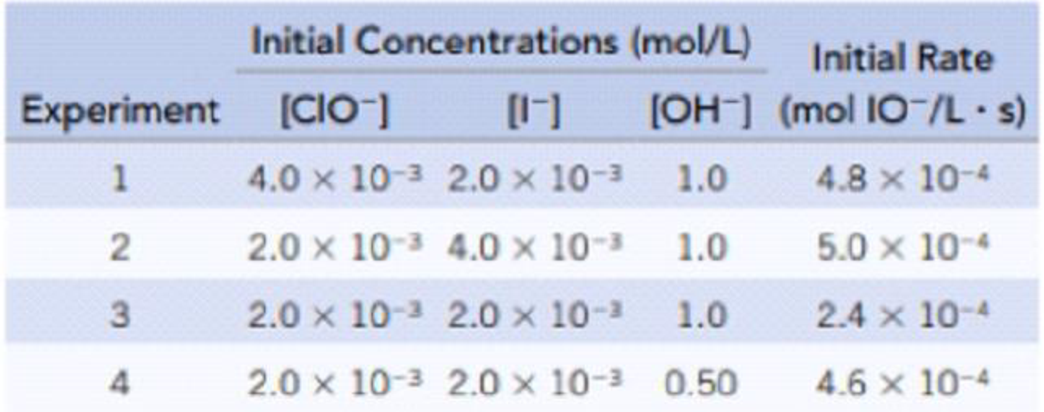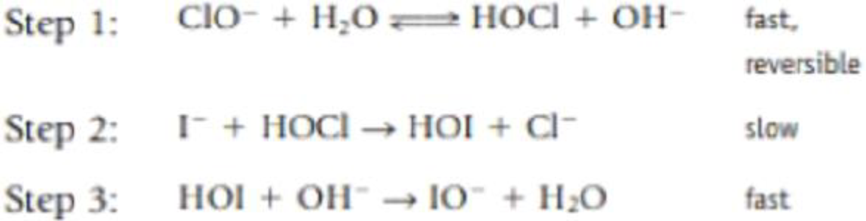Chapter 14, Problem 89IL

Chapter
Section
Textbook Problem

The oxidation of iodide ion by the hypochlorite ion in the presence of hydroxide ionsI−(aq) + ClO−(aq) → IO−(aq) + Cl−(aq)was studied at 25 °C, and the following initial rates data (Y. Chia and R. E. Connick, Journal of Physical Chemistry, Vol. 63, p. 1518, 1959) were collected:(a) Determine the rate law for this reaction. (b) One mechanism that has been proposed for this reaction is the following:Show that the rate law predicted by this mechanism matches the experimentally determined rate law in part a. (Note that when writing the expression for K the equilibrium constant, [H2O] is not involved. See Chapter 15.)

(a)

Interpretation Introduction

Interpretation:

The rate law of the given reaction should be given.

Concept Introduction:

Rate law: It is generally the rate equation that consists of the reaction rate with the concentration or the pressures of the reactants and constant parameters.

Rate constant: The rate constant for a chemical reaction is the proportionality term in the chemical reaction rate law which gives the relationship between the rate and the concentration of the reactant present in the chemical reaction.

Explanation

The rate law is obtained by first determining the order of each reactant present in the given reaction. The order of each reactant is obtained by using the given set of concentration and the rate data as follows,

The order for ClO is obtained by considering the experiment 1 and 3 from the given data.

13isasfollows,[ClO]1[ClO]3[I]1[I]3[OH]1[OH]3=[Rate]1[Rate]34×10-32×10-31.04.8×10-42×10-32×10-31.02.3×10-4 _2m21

The order for ClO is 1

(b)

Interpretation Introduction

Interpretation:

The rate law predicted by the mechanism matches the experimentally determined rate law should be shown.

Concept Introduction:

Rate order: The order of each reactant in a reaction is represented by the exponential term of the respective reactant present in the rate law and the overall order of the reaction is the sum of all the exponents of all reactants present in the chemical reaction. The order of the reaction is directly proportional to the concentration of the reactants.

Rate law: It is generally the rate equation that consists of the reaction rate with the concentration or the pressures of the reactants and constant parameters.

Rate constant: The rate constant for a chemical reaction is the proportionality term in the chemical reaction rate law which gives the relationship between the rate and the concentration of the reactant present in the chemical reaction.

Still sussing out bartleby?

Check out a sample textbook solution.

See a sample solution

The Solution to Your Study Problems

Bartleby provides explanations to thousands of textbook problems written by our experts, many with advanced degrees!

Get Started

What are the moral implications of using ergogenic aids?

Understanding Nutrition (MindTap Course List)

• define the term orbital.

Chemistry for Engineering Students

What energy sources on Earth cannot be thought of as stored sunlight?

Horizons: Exploring the Universe (MindTap Course List)

Does the cell cycle refer to mitosis as well as meiosis?

Human Heredity: Principles and Issues (MindTap Course List)

Where is most fluid in the body located? What is it called?

Chemistry for Today: General, Organic, and Biochemistry

Match the term listed in Column A with its definition from Column B

Nutrition Through the Life Cycle (MindTap Course List)